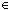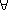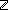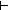IF YOU CAN SEE THIS go to /sfa/Nuprl/Shared/Xindentation_hack_doc.html

### Type Functionality Sequents and Level Expressions - from Functionality Sequents and Universes

Discussions in type theory often refer to expressions being "functional" on some input types and with respect to some output type (see Functions). But also we use the concept of an expression being "type-functional" on some input types, meaning equal inputs give output expressions denoting the same type. As was mentioned in Universes, we cannot assimilate the concept of type-functionality to functionality with respect to an output type because there is no type TYPE comprising all types; if there were, then type-functionality would simply be functionality with respect to output type TYPE.

Although reasoning about type functionality via a type of all types is impossible, one might still reason about it by adding a form of assertion about it, as Martin-Lof did in his 1980 type system from which Nuprl stems. Despite the advantages of reasoning about type-functionality explicitly, the original designers of Nuprl hoped to simplify their design by not doing so (however, such rules are likely to be incorporated eventually); rather, the primitive rules of Nuprl simply approximate most reasoning about "all types" by reasoning instead about a hierarchy of types Type{i} called "universes", which together cover all types (see Universes).

So, rather than having a rule saying, for example, that A+B is a type if A and B are, there is a rule that allows us to infer that A+BType{i} given AType{i} and BType{i}, whatever i might be. Indeed one can prove Thm*A,B:Type{i}. A+BType{i}.

Notice that in the statement of Thm*A,B:Type{i}. A+BType{i}, the universe level is left as a variable rather than a constant. In a Nuprl, the meaning of a sequent is always general with respect to choices of levels that can be assigned to letters used inside universe level expressions.

Consider Thm*A:Type{i}, B:Type{j}. A+BType{[i | j]}.
This says that the disjoint union of two types from any two universe levels is a type in the universe whose level is the maximum of the two levels. So, there is a "max" operation you can use in universe level expressions.

There is also a successor operation: Thm* Type{i}Type{i'}.
As a convenience, there is also an offset operation that adds a constant to a level: Thm* Type{i'}Type{i 2}.

Although there is no need to do so in a theorem statement, one can also use constant level expressions: Thm*Type{3}.

The forms of level expression may be freely combined.

Universe Polymorphism.

The reason it is practical to use these variables in level expressions (but note that they are not like other ordinary variables of the logic -- you cannot bind them) is that any true sequent remains true if all the level variables throughout it are uniformly replaced by level expressions of your choice.So from "A:Type{i}, B:Type{j}. A+BType{[i | j]}" it follows that "A:Type{3}, B:Type{k'}. A+BType{[3 | k']}".
It also follows that "A,B:Type{i}. A+BType{[i | i]}" which is equivalent to "A,B:Type{i}. A+BType{i}".

In a typical sequent, it turns out, all the universe level expressions are the same and consist of simply a level variable. When this is so, we normally choose to display the universe expression simply as "Type" rather than in full, such as "Type{i}". Thus, one would normally display the sequent "A,B:Type{i}. A+BType{i}" simply as "A,B:Type. A+BType".

The same level expressions are used as indices to Prop{[level]}. See Prop Levels and Predicativity.

(Feb 2001 - sfa )